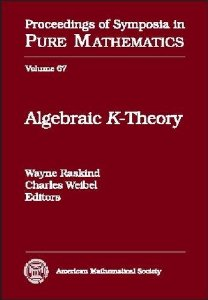## Series Monográficas: Proceedings of symposia in pure mathematicsVol. 47. Fong, Paul (Ed.)
The Arcata Conference on Representations of Finite Groups. Part 1 and Part 2.
QA171 A58

Vol. 23. Spence, Donald Clayton (Ed.)

QA374 S92 1971

Vol. 46. Bloch, Spencer; Clemens, Charles Herbert (Eds.)
Algebraic geometry : Bowdoin 1985. Part 1 and Part 2.
QA564 S85 1985

Vol. 22. Liulevicius, Arunas (Ed.)
Algebraic topology
QA612 S942 1970

Vol. 45. Browder, Felix E., (Ed.)
Nonlinear functional analysis and its applications. Part 1 and Part 2.
QA321.5 N65

Vol. 21. Reiner, Irving (Ed.)
Representation theory of finite groups and related topics
QA171 S913 1970

Vol. 44. Amgren, Frederick J.; Allard, William K. (Eds.)

QA312 G46

Vol. 20. Lewis, donald J. (Ed.)
1969 number theory institute
QA241 N826

Vol. 43. Treves, Francois (Ed.)

QA329.7 S95 1984

Vol. 19. Motzkin, Theodore Samuel (Ed.)

QA164 S94

Vol. 42. Nerode, Anil; Shore, Richard A. (Eds.)
Recursion theory
QA9.6 R44

Vol. 41. Siu, Yum-Tong (Ed.)
Complex analysis of several variables
QA331 C644

Vol. 17. Heller, Alex (Ed.)
Applications of categorical algebra
QA169 S94

Vol. 40. Orlik, Peter (Ed.)
Singularities. Part 1 and Part 2.
QA614.58 S84 1981

Vol. 16. Chern, Shiing-Shon; Smale Stephen (Eds.)
Global analysis
QA614 S9 1968

Vol. 39. Browder, Felix E., (Ed.)
The mathematical heritage of Henri Poincare. Part 1 and Part 2.
QA1 M37

Vol. 15. Chern, Shiing-Shon; Smale Stephen (Eds.)
Global analysis
QA614 S9 1968

Vol. 38. Kadison, Richard V. (Ed.)
Operator algebras and applications. Part 1 and Part 2.
QA326 S94 1980

Vol. 14. Chern, Shiing-Shen; Smale, Stephen (Eds.)

QA614 S9 1968

Vol. 37. Cooperstein, Bruce; Mason, Geoffrey (Eds.)
The Santa Cruz Conference on Finite Groups
QA171 S945 1979

Vol. 13. Scott, Dana S. (Ed.)
Axiomatic set theory. Part 1 and Part 2.
QA248 S94 1967

Vol. 36. Osserman, Robert ; Weinstein, Alan  (Eds.)

QA614 A57 1979

Vol. 12. Leveque, Willia, Judson; Straus, Ernest G. (Eds.)
Number theory
QA241 N81

Vol. 35. Weiss, Guido; Wainger, Stephen (Eds.)
Harmonic analysis in euclidean spaces. Part 1 and Part 2.
QA403 S94 1978

Vol. 11. Korevaar, Jacob; Chern, S. S.;  Ehrenpreis, Leon; Fuchs, W. H. J.; Rubel, L. A. (Eds.)
Entire functions and related parts of analysis
QA351 S92 1966

Vol. 34. Ray-chaudhuri, Dijen K. (Ed.)
Relations between combinatorics and other parts of mathematics
QA164 S94 1978

Vol. 10. Calderon, Alberto P. (Ed.)
Singular integrals
QA311 S93 1966

Vol. 33. Borel, Armand; Casselman, W. (Eds.)
Automorphic forms, representations and L-functions : proceedings of the Symposium in Pure Mathematics of the American Mathematical Society, held at Oregon State University, Corvallis, Oregon, July II-August 5, 1977. Part 1 and Part 2.
QA331 S92 1977

Vol. 9. Borel, Armand; Mostow, George (Eds.)
Algebraic groups and discontinuous subgroups
QA171 A52

Vol. 32. Milgram, R. James (Ed.)
Algebraic and geometric topology. Part 1 and Part 2.
QA612 S94 1976

Vol. 8. Whiteman, Albert Leon (Ed.)
Theory of numbers
QA241 S9

Vol. 31. Doob, Joseph L. (Ed.)
Probability
QA273.A1 S96 1976

Vol. 30. Wells, Raymond O'neil (Ed.)
Several complex variables. Part 1 and  Part 2.
QA331 S88 1975

Vol. 5. Dekker, J. C. E. (Ed.)
Recursive function theory
QA248.5 S9

Vol. 28. Browder, Felix E. (Ed.)
Mathematical developments arising from Hilbert problems. Part 1 and Part 2.
QA1 S97 1974

Vol. 4. Morrey, Charles Bradfield (Ed.)
Partial differential equations
QA374 S9

Vol. 27. Chern, Shiing-Shen; Osserman, R. (Eds.)
Differential geometry. Proceedings of the Symposium in Pure Mathematics of the American Mathematical Society, held at Stanford University, Stanford California, july 30-august 17, 1973. Part 1 and Part 2.
QA641 S95 1973

Vol. 24. Diamond, Harold G. (Ed.)
Analytic number theory
QA241 S92 1972

### ¿Quién está en línea?

Hay 78 invitados y ningún miembro en línea

Go to top